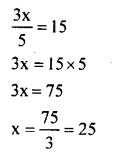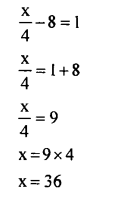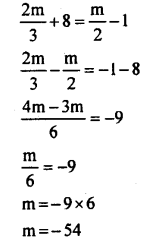# KSEEB Solutions for Class 8 Maths Chapter 8 Linear Equations in One Variable Ex 8.1

Students can Download Maths Chapter 8 Linear Equations in One Variable Ex 8.1 Questions and Answers, Notes Pdf, KSEEB Solutions for Class 8 Maths helps you to revise the complete Karnataka State Board Syllabus and score more marks in your examinations.

## Karnataka Board Class 8 Maths Chapter 8 Linear Equations in One Variable Ex 8.1

1. Solve the following:

Question i.
x + 3 = 11
x + 3 = 11
x = 11 – 3
x = 8

Question ii.
y – 9 = 21
y – 9 = 21
y = 21 + 9
y = 30

Question iii.
10 = z + 3
10 = z + 3 10-3 =z
7 = z or z = 7

Question iv.
$$\frac{3}{11}+x=\frac{9}{11}$$Question v.
10x = 30
10x = 30
x = $$\frac { 30 }{ 10 }$$
x = 3

Question vi.
$$\frac{s}{7}=4$$
$$\frac{s}{7}=4$$
S = 4 × 7
S = 28

Question vii.
$$\frac{3 x}{6}=10$$
$$\frac{3 x}{6}=10$$
3x = 10 × 6
3x = 60
x = $$\frac { 60 }{ 3 }$$
x = 20

Question viii.
$$1.6=\frac{x}{1.5}$$
$$1.6=\frac{x}{1.5}$$
1.6 × 1.5 = x
2.40 = x
x = 2.4

Question ix.
8x – 8 = 48
8x – 8 = 48
8x = 48 + 8
8x = 56
x = $$\frac { 56 }{ 8 }$$
x = 7

Question x.
$$\frac{x}{3}+1=\frac{7}{15}$$Question xi.
$$\frac{x}{5}=12$$
$$\frac{x}{5}=12$$
x = 12 × 5
x = 60

Question xii.
$$\frac{3 x}{5}=15$$Question xiii.
3(x + 6) = 24
3(x + 6) = 24
3x+ 18 = 24
3x = 24 – 18
3x = 6
x = $$\frac { 6 }{ 3 }$$ = 2

Question xiv.
$$\frac{x}{4}-8=1$$Question xv.
3(x+2) – 2(x—1) = 7
3(x + 2) – 2(x – 1) = 7
3x + 6 – 2x + 2 = 7
x + 8 = 7 .
x = 7 – 8
x = -1

2. Solve the equations :

Question i.
5x = 3x + 24
5x = 3x + 24
2x = 24
x = $$\frac { 24 }{ 2 }$$
x = 12

Question ii.
8t + 5 = 2t – 31
8t + 5 = 2t – 31
6t = -36
t = $$\frac { -36 }{ 6 }$$
t = -6

Question iii.
7x -10 = 4x + 11
7x – 10 = 4x + 11
7x – 4x = 11 + 10
3x = 21
x = $$\frac { 21 }{ 3}$$
x = 7

Question iv.
4z + 3 = 6 + 2z
4z + 3 = 6 + 2z
4z – 2z = 6 – 3
2z = 3

Question v.
2x – 1 = 14 – x
2x – 1 = 14 – x
2x + x = 14 + 1
3x = 15
x = $$\frac { 15 }{ 3 }$$
x = 5

Question vi.
6x + 1 = 3(x -1) + 7
6x + 1 = 3(x —1) + 7
6x + l =3x-3 + 7
6x + l = 3x + 4
6x – 3x = 4 – 1
3x = 3
x = $$\frac { 3 }{ 3 }$$
x = 1

Question vii.
$$\frac{2 x}{5}-\frac{3}{2}=\frac{x}{2}+1$$Question viii.
$$\frac{x-3}{5}-2=\frac{2 x}{5}$$Question ix.
3(x + 1) = 12 + 4(x – 1)
3(x + 1)= 12 + 4(x – 1)
3x + 3 = 12 + 4x – 4
3x + 3 = 4x + 8
3x – 4x = 8 – 3
-x = 5
x = -5

Question x.
2x – 5 = 3(x – 5)
2x – 5 = 3(x – 5)
2x – 5 = 3x – 15
2x – 3x = -15 + 5
-x = -10
x= 10

Question xi.
6(1 – 4x) + 7(2 + 5x) = 53
6( 1 – 4x) + 7(2 + 5x) = 53
6 – 24x+ 14 + 35x = 53
35x – 24x + 6 + 14 = 53
11x+ 20 = 53
11x = 53 – 20
x = $$\frac { 33 }{ 11 }$$
x = 3

Question xii.
3(x + 6) + 2(x + 3) = 64
3(x + 6) + 2(x + 3) = 64
3x + 18 + 2x + 6 = 64
3x + 2x + 18 + 6 = 64
5x + 24 = 64
5x = 64 – 24
5x = 40
x = $$\frac { 40 }{ 5 }$$
∴ x = 8

Question xiii.
$$\frac{2 m}{3}+8=\frac{m}{2}-1$$$$\frac{3}{4}(x-1)=(x-3)$$
$$\frac{3}{4}(x-1)=(x-3)$$